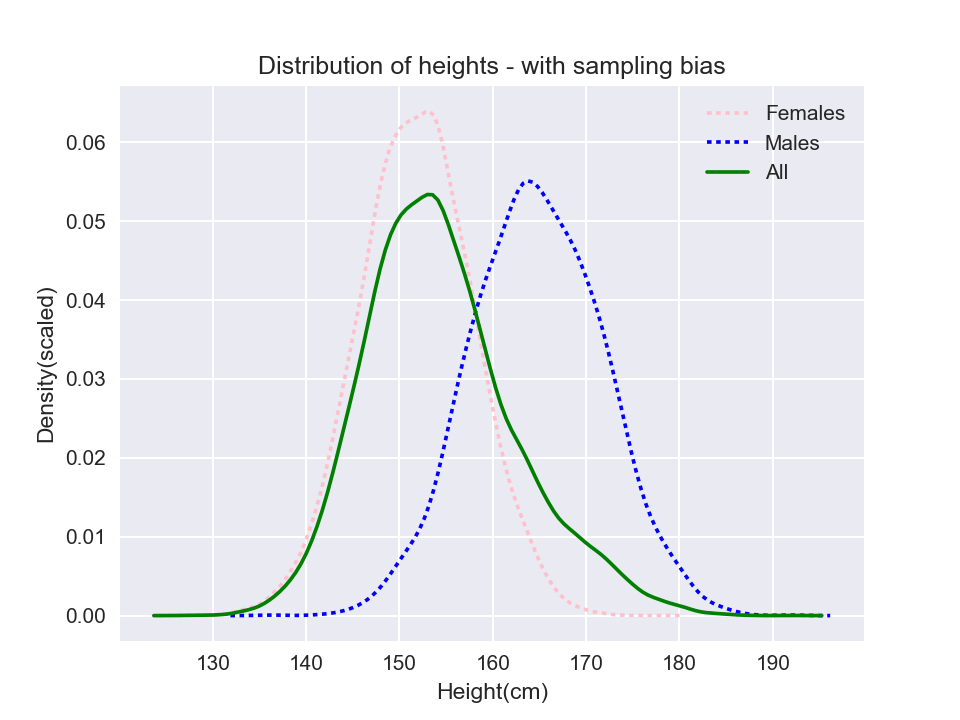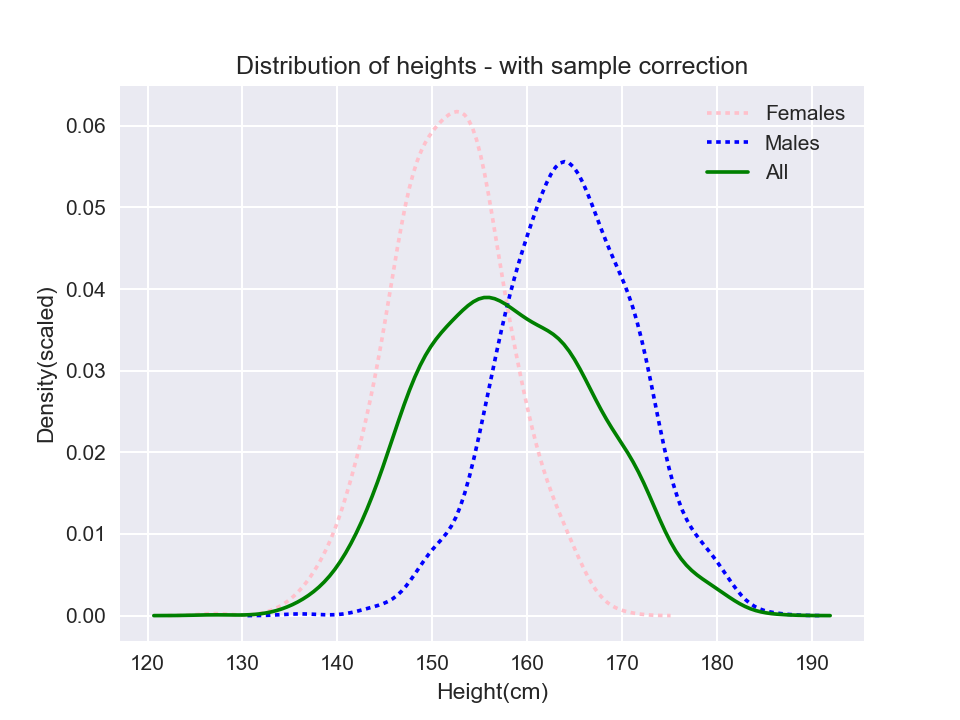Let’s say we wanted to find the average height of an Indian. We embark on a survey and interview people on their heights. During the process, we just happened to interview a lot more females than males. This would result in a selection that is not truly representative of the actual population. If we were to find the average height from this sample; we would probably get a smaller height than expected, considering that the average female height is lesser.

One would expect an approximate 50:50 ratio of males and females in the sample, discounting the horrible skewed sex ratio in India (940 females for 1000 males is how it stands currently!).

We’ve unintentionally introduced a sampling bias, by biasing the selection towards females. A sampling bias is introduced when one were to collect a sample in a non-random, non-distributed way. The results from such samples are often skewed and factually inaccurate.

Now for some code. We’ll use the adorable `pandas` and `numpy` libraries from the Python world. Let’s work backwards from the following (questionable) facts:

Assume we interviewed 16000 females and 4000 males and got the following results:

``````import pandas as pd
import numpy as np
import matplotlib.pyplot as plt
import seaborn as sns
import warnings
warnings.filterwarnings('ignore')

%matplotlib notebook

female_heights = np.random.normal(loc = 151.9, scale = 6, size = 16000)
male_heights = np.random.normal(loc = 164.9, scale = 7, size = 4000)

all_heights = np.append(female_heights, male_heights)
all_genders = ['F'] * 16000 + ['M'] * 4000

df = pd.DataFrame({'Gender': all_genders, 'Height': all_heights})
``````
Gender Height
0 F 161.321140
1 F 155.368424
2 F 148.209417
3 F 165.482523
4 F 144.688549

Let’s plot the height distributions:

``````plt.figure()
sns.distplot(pd.Series(female_heights, name = "Height(cm)"), hist = False, label = "Females",
color='pink', kde_kws={'linestyle': 'dotted'})
sns.distplot(pd.Series(male_heights, name = "Height(cm)"), hist = False, label = "Males",
color = 'blue', kde_kws={'linestyle': 'dotted'})
sns.distplot(all_heights, hist = False, label = "All",
color = 'green')

plt.gca().set_ylabel('Density(scaled)')
plt.gca().set_title('Distribution of heights - with sampling bias')
plt.legend();
``````
``````<IPython.core.display.Javascript object>
``````The above plot presents a glaring error: the ‘All’ distribution follows the ‘Female’ distribution more closely than it should have. It is clear that we have to somehow ‘weight’ the observations. If entries for males can be assigned a weight of `1`, entries for females should have `4000/16000` - i.e. (number of males/number of females) = `0.25`:

``````def set_weight(row):
if row['Gender'] == 'F':
row['Weight'] = 0.25
else:
row['Weight'] = 1
return row

df_with_weights = df.apply(set_weight, axis = 1)
``````
Gender Height Weight
0 F 161.321140 0.25
1 F 155.368424 0.25
2 F 148.209417 0.25
3 F 165.482523 0.25
4 F 144.688549 0.25

The pandas `DataFrame` provides a `sample` method, where we can extract a sub-sample from our original data frame. This would yield us a dataframe which would be more representative of our original population. Let’s extract a sample of size 2000:

``````sample = df_with_weights.sample(n=2000, weights='Weight')
print('Number of males: ', sample[sample.Gender == 'M'].shape)
print('Number of females: ', sample[sample.Gender == 'F'].shape)
``````
``````Number of males:  1003
Number of females:  997
``````

The above seems to represent the gender ratio more realistically. The distribution for the sample distribution looks like:

``````plt.figure()
sns.distplot(pd.Series(sample[sample.Gender == 'F'].Height, name = "Height(cm)"), hist = False, label = "Females",
color='pink', kde_kws={'linestyle': 'dotted'})
sns.distplot(pd.Series(sample[sample.Gender == 'M'].Height, name = "Height(cm)"), hist = False, label = "Males",
color = 'blue', kde_kws={'linestyle': 'dotted'})
sns.distplot(sample.Height, hist = False, label = "All",
color = 'green')

plt.gca().set_xlabel('Height(cm)')
plt.gca().set_ylabel('Density(scaled)')
plt.gca().set_title('Distribution of heights - with sample correction')
plt.legend();
``````
``````<IPython.core.display.Javascript object>
``````Although we considered only ‘Gender’, several other variables could have contributed to sampling bias such as ‘Age’, ‘State’ (heights vary from one Indian state to another partly due to socio-economic factors). The ‘Weight’ that we assign to each observation should therefore consider each of the above factors into account.

Another good example of sampling bias were the US election surveys that used landlines to contact participants. This was not a problem for a long time. However after the turn of this century, an increasing number of homes have become cell-only: especially with people under 30. By not reaching out to cell-phones, some age groups could be under-represented.